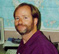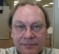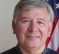## Upsampling of dataStarted by 6 years ago8 replieslatest reply 6 years ago377 views

Hi,

I need to be able to convert a set of data composed of X samples with amplitude Y to a new set of data.

The original data has anywhere from 1024 samples to 2048 samples.

I want to convert the data so that I always have 2048 samples.

I have no problems converting from 1024 to 2048 but what about other arbitrary values.  Say 1279 to 2048?  How does one go about this?  This is purely for plotting purposes.

Thanks for any hints.  Tom

[ - ]Linear interpolation is probably fine for this.  Loop through each of the 2048 values you want to calculate in the converted data, say, x = 1 to 2048.  Then for each point calculate the fractional index in the original data  p = x * 1279/2048.  That is, p resides between two samples in your input data.  Just interpolate between those samples to get the value in the converted data.

[ - ]There are lots of ways to do this, including linear interpolation, spline interpolation, etc.   Also look into "multirate filtering" or similar topics such as polyphase filtering.   As usual, each method has some tradeoff relating to the types of distortion that they may or may not cause, as well as the level of complexity in the computation, etc., etc.   What works for other applications may not work for yours depending on your sensitivity to the various potential distortions, etc.

You can even do something like use DFTs with zero padding, e.g., take the DFT of the length N array, zero pad it up to 2048, take the inverse DFT back to the original domain.

[ - ]You can even do something like use DFTs with zero padding, e.g., take the DFT of the length N array, zero pad it up to 2048, take the inverse DFT back to the original domain.

If you're working in something like Scilab or Matlab, this is a good way to go.  There'll probably be funny end effects, and wanting to get the whole thing kinda implies that windowing is out of the question.

[ - ]tomb18: Just think about what you just said as you described your "problem". This sounds like an ordinary case of interpolation, which when I went to school in the late 50's to early 70's was introduced somewhere around 8th grade ('64-65) as part of math and continued to get more involved as time went on. It never advanced much beyond linear interpolation, since for the most part, it doesn't typically need to be more accurate than that. What screen resolution is your goal? What if you reversed what you said? Instead of making 2048 the goal, make 1024 the goal. Would the interpolation be much different? There are lots of algorithms out there to doing a "better" job than simple linear interpolation, but they tend to be specific to some desired effect (e.g. not lose "high frequency" content, or perhaps to make it less "noisy"). Is your data adequately sampled (e.g. Nyquist considerations)? And know that our eyes can only handle so much data before they fog over from too much detail. Prototype some versions and see if they meet your desires. There are also statistical data packages out there that have lots of plotting options. Even GNUplot has many and it's free.

A great series of books for off-the-shelf algorithmic answers is any of the "Numerical Recipes in X" where "X" is the programming language (I only personally have the older and original C version from 1988 that I got from a friend when he got a newer edition). Apparently they have a web site now: http://numerical.recipes/.

Happy hunting.

[ - ]Thanks for all the answers. yes linear interpolation is fine. I guess what through me off was the cases like starting with 2047 samples and interpolating to 2048....

I absolutely need 2048 samples.  Using less is much more complex in this case.

Basically what I am doing is plotting in real time a 3D spectrum.  It is basically a "heat map" of 2048 x 100 points. Supplying 2048 points is the way to go vs changing the heat map as one zooms in the source.

Thanks, I should be good to go once I figure out the 2047 to 2048...

[ - ]For graphics purposes linear (or more generally polynomial or spline) interpolation is fine.

However, it is a really BAD idea to use polynomial interpolation for most signal processing purposes, since it severely distorts the signal's spectrum, and specifically adds spectral components above the original Nyquist frequency.

For signals you need first to decide whether your application requires to keep the overall time duration and to resample (in which case the low-pass sampling theorem gives a closed formula for the value of a signal at any time) or whether the time duration is correct for the original number of samples and you want to extend the duration (in which case AR prediction may be the correct answer).

Y(J)S

[ - ]I agree with everyone and, particularly, with Tim.  The "edge" effects may be a real issue (or not).

Here is a classical approach to time-domain interpolation using an FFT/IFFT.

1) FFT the time samples.  The resulting frequency samples will NOT necessarily be zero or have a double zero at fs/2.

2) Insert zeros symmetrically at fs/2 so you end up with 2048 samples.  So 2048-1279= 769 zeros to insert.  Being an odd number, one of the samples will be at fs/2.  The problem with doing this is if the samples you start with around the original fs/2 are nonzero.  Ideally, the samples would have a double zero at fs/2 so the slope is also zero.  So...

3) lowpass filter the data - well, in this case perhaps, notch filter the data at fs/2 so that there is a double zero at fs/2.  This is what half-band filters are often used for.  The end result in that case is that you end up with almost all the data but with the sample rate doubled.  So this would work for your 1024 case.

4) Once the data is filtered, you can insert zero-valued samples without introducing sharp transitions at the edges of the sequence of zeros in the resulting data.

5) Then, of course, if you were needing higher rates of sample-rate increase, you could insert as many zeros as you like - without introducing sharp edges and without repeated applications of a half-band filter.

[ - ]I read the answers to the posts and cannot resist to add this reply even though I think the methods below will solve your specific problem well enough.

I am a little surprised that for a question that has a defined and relatively simple answer in signal theory you get so many replies suggesting simplifications or approximations of that answer and so I thought, I should add (what I assume to be) the theoretically correct solution.

Your original signal has samplerate Fs1 (=1279 in your example) and you want to convert to Fs2 (= 2048).

1. Upsample your signal to Fsnew = Fs1*Fs2 by inserting Fs2 zeros after every original sample.

2. Low-Pass filter the result by a low pass with normalized cut-off Fs1 / (2*Fs1*Fs2) (unnormalized cut-off = Fs1/2).

3. Downsample the result by Fs1

And you have your result. This may seem like a whole lot of effort - filtering a signal at a samplerate of 2.6M instead of 2.048k - but it is not. Since this filter can be FIR, you only need to calculate it for those samples that you actually keep in the downsampling step 3. Furthermore, most of the input to the FIR is zero (remember putting in Fs2 zeros between two input samples). So you end up with relatively few calculations you have to perform Fs2 times.

This method is optimal considering the sampling theorem - you will keep all frequencies present in your orignial signal without distortion and add no frequencies above... It may not be optimal in some other sense...